Courses

# CDS I - Mathematics Question Paper 2019

## 100 Questions MCQ Test CDS (Combined Defence Services) Previous Years Papers | CDS I - Mathematics Question Paper 2019

Description
This mock test of CDS I - Mathematics Question Paper 2019 for Defence helps you for every Defence entrance exam. This contains 100 Multiple Choice Questions for Defence CDS I - Mathematics Question Paper 2019 (mcq) to study with solutions a complete question bank. The solved questions answers in this CDS I - Mathematics Question Paper 2019 quiz give you a good mix of easy questions and tough questions. Defence students definitely take this CDS I - Mathematics Question Paper 2019 exercise for a better result in the exam. You can find other CDS I - Mathematics Question Paper 2019 extra questions, long questions & short questions for Defence on EduRev as well by searching above.
QUESTION: 1

### Direction: Consider the following for the next three (03) items : A cube is inscribed in a sphere. A right circular cylinder is within the cube touching all the vertical faces. A right circular once is inside the cylinder. Their heights are same and the diameter of the cone is equal to that of the cylinder. Q. What is the ratio of the volume of the sphere to that of cone?

Solution: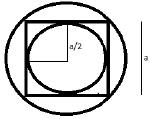The top view of the given assembly will look like the figure above
Outermost is the sphere. Inside that there is a cube and within that there is a cone and cylinder with same radius.
Here side of cube = a
Diameter of Sphere = body diagnol = √3 a
Radius of sphere = √3 a/2 = r1
Height of Cylinder = Height of cone = side of cube = a = h
Radius of cylinder = Radius of cone = side of cube/2 = a/2 = r2 (as shown in the figure)
Volume of sphere/volume of cone =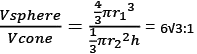QUESTION: 2

### Direction: Consider the following for the next three (03) items : A cube is inscribed in a sphere. A right circular cylinder is within the cube touching all the vertical faces. A right circular once is inside the cylinder. Their heights are same and the diameter of the cone is equal to that of the cylinder.​ Q. What is the ratio of the volume of the cube to that of the cylinder ?

Solution: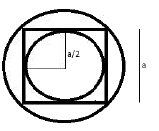The top view of the given assembly will look like the figure above
Outermost is the sphere. Inside that there is a cube and within that there is a cone and cylinder with same radius.
Here side of cube = a Diameter of Sphere = body diagnol = √3 a
Radius of sphere = √3 a/2 = r1
Height of Cylinder = Height of cone = side of cube = a = h
Radius of cylinder = Radius of cone = side of cube/2 = a/2 = r2 (as shown in the figure)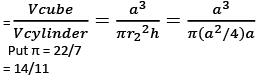QUESTION: 3

### Direction: Consider the following for the next three (03) items : A cube is inscribed in a sphere. A right circular cylinder is within the cube touching all the vertical faces. A right circular once is inside the cylinder. Their heights are same and the diameter of the cone is equal to that of the cylinder. 1) The surface area of the sphere is √5 Times the curved surface area of the cone. 2) The surface area of the cube is equal to the curved surface area of the cylinder. Q. Which of the above statements is/are correct?

Solution: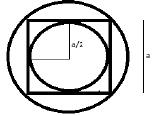The top view of the given assembly will look like the figure above
Outermost is the sphere. Inside that there is a cube and within that there is a cone and cylinder with same radius.
Here side of cube = a
Diameter of Sphere = body diagnol = √3 a
Radius of sphere = √3 a/2 = r1
Height of Cylinder = Height of cone = side of cube = a = h
Radius of cylinder = Radius of cone = side of cube/2 = a/2 = r2 (as shown in the figure)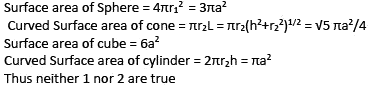QUESTION: 4

Direction: Consider the following for the next three (03) items:
ABCD is a quadrilateral with AB = 9 cm, BC = 40 cm, CD = 28 cm, DA = 15 cm and angle ABC is a right – angel
Q. What is the area of triangle ADC?

Solution: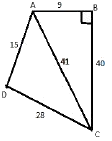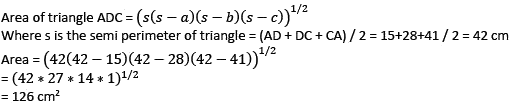QUESTION: 5

Direction: Consider the following for the next three (03) items:
ABCD is a quadrilateral with AB = 9 cm, BC = 40 cm, CD = 28 cm, DA = 15 cm and angle ABC is a right – angel
Q. What is the area of quadrilateral ABCD?

Solution: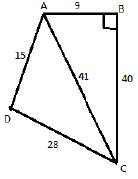Area of quadrilateral ABCD = area of triangle ADC + area of triangle ABC
= 126 + ½ * 9 * 40 = 306 cm2

QUESTION: 6

Direction: Consider the following for the next three (03) items:
ABCD is a quadrilateral with AB = 9 cm, BC = 40 cm, CD = 28 cm, DA = 15 cm and angle ABC is a right – angel
Q. What is the difference between perimeter of triangle ABC and perimeter of triangle ADC?

Solution: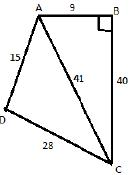Perimeter of triangle ABC – Perimeter of triangle ADC = (9+40+41)-(15+28+41) = 6cm

QUESTION: 7

Direction: Consider the following for the next two (02) items:
An equilateral triangle ABC is inscribed in a circle of radius 20√3
Q. What is the length of the side of the triangle?

Solution: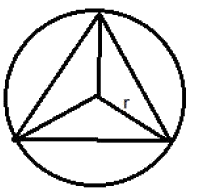Radius of circumcircle of an equilateral triangle = side / √3
R = a/√3
a = R√3  = 20√3  * √3  = 60cm

QUESTION: 8

Direction: Consider the following for the next two (02) items:
An equilateral triangle ABC is inscribed in a circle of radius 20√3
Q. The centroid of the triangle ABC is at a distance d from the vertex A. What is d equal to?

Solution: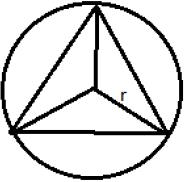For equilateral triangle circumcenter and centroid are the same points
So distance from vertex = radius of circumcircle = 20√3

QUESTION: 9

Direction: Consider the following for the next two (02) items:
The sum of length, breadth and height of a cuboid is 22 cm and the length of its diagonal is 14 cm.
Q. What is the surface area of the cuboid?

Solution:

Let lengths, breadth and height of cuboid be l, b and h respectively
According to question l+b+h = 22cm……(i)
and √(l2+b2+h2) = 14cm …..(ii)
Surface area of cuboid = 2(lb+bh+lh)
Squaring eq (i) gives
l2+b2+h2 + 2(lb+bh+lh) = 484
Substituting l2+b2+h2 from eq (i)
2(lb+bh+lh) = 484-196 = 288 cm2

QUESTION: 10

Direction: Consider the following for the next two (02) items:
The sum of length, breadth and height of a cuboid is 22 cm and the length of its diagonal is 14 cm.
Q. If S is sum of the cubes of the dimensions of the cuboid and V is its volume, then what is (S-3V) equal to?

Solution:

Let lengths, breadth and height of cuboid be l, b and h respectively
According to question
l+b+h = 22cm……(i)
and √(l2+b2+h2) = 14cm …..(ii)
S = l3+b3+h3 and  V = lbh
S-3V = l3+b3+h3 - 3 lbh = (l+b+h)( l2+b2+h2-[lb+bh+lh])…(iii)
As we know
Squaring eq (i) gives
l2+b2+h2 + 2(lb+bh+lh) = 484
Substituting l2+b2+h2 from eq (i)
2(lb+bh+lh) = 484-196 = 288 cm2
lb+bh+lh = 144 cm2
Putting this in eq (iii) we get
22(196-144) = 22*52 = 1144cm2

QUESTION: 11

A race has three parts. The speed and time required to complete the individual parts for a runner is displayed on the following chart:​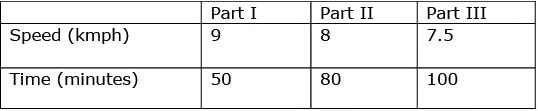Q. What is the average speed of this runner?

Solution:

Average speed = Total Distance / Total time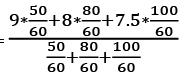= (45+64+75)/23 = 184/23
= 8 kmph

QUESTION: 12

If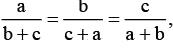then which one of the following statements is correct?

Solution:

a/(b+c) = b/(c+a) = c/(a+b)
Taking reciprocal and adding 1 to each ratio we get;
(b+c)/a + 1 = b/(c+a) + 1 = c/(a+b) + 1
Or (a+b+c)/a = (a+b+c)/b = (a+b+c)/c
So this can only be equal when a=b=c or a+b+c = 0
When a=b=c we get a/(b+c) = ½
When a+b+c = 0 we get b+c = -a
So a/(b+c) = -1
So the ratios are ½ or -1

QUESTION: 13

The number 3521 is divided by 8. What is the remainder?

Solution:

3521/8
As we know 32=9 will leave remainder = 1 when divided by 8
So 3521/8 = [(32)260 * 3]/8 = 1*3/8 = 3/8 Thus remainder is 3

QUESTION: 14

A prime number contains the digit X at unit’s place. How many such digits of X are possible?

Solution:

For prime no units place cannot be occupied by even number except for 2 Thus no of digits occupying unit digit of prime numbers = 6 (1,2,3,5,7,9) Example 2,3,5,7,11,19 in itself are prime numbers

QUESTION: 15

If an article is sold at a gain of 6% instead of a loss of 6% the seller gets Rs. 6 more. What is the cost price of the article?

Solution:

Let CP be Rs x
Then
1.06x – 0.94x = 6
So x = Rs 50

QUESTION: 16

A field can be reaped by 12 men or 18 women in 14 days. In how many days can 8 men and 16 women reap it?

Solution:

12 men or 18 women can complete in 14 days
8 men and 16 women can complete in how many days
12men = 18 women (Comparing efficiencies)
1men = 18/12 = 1.5 women
8 men and 16 women = 12women + 16 women = 28 women
18 women completes in 14 days
1 woman completes in 14*18 days
28 women completes in (14*18)/28 days = 9 days

QUESTION: 17

If 3x = 4y = 12z, then z is equal to

Solution:

3x = 4y = 12z
Taking log of all 3 we get
xln3 = yln4 = zln12 = k
z = k/ln12 = k / ln(3*4) = k/ln3 + ln4 = k / (k/x +k/y) = xy / (x+y)

QUESTION: 18

If (4a +7b) (4c-7d) = (4a -7b) (4c +7d, then which one of the following is correct?

Solution:

(4a+7b)(4c-7d) = (4a-7b)(4c+7d)
(4a+7b)/(4a-7b) = (4c+7d)/(4c-7d)
Using componendo and dividendo
(4a+7b)+(4a-7b) / (4a+7b)-(4a-7b) = (4c+7d)+(4c-7d) / (4c+7d)-(4c-7d)
Or 8a/14b = 8c/14d
Or a/b = c/d

QUESTION: 19

GiGiven that the polynomial (x2 + ax + b) leaves that same remainder when by (x – 1) or (x + 1) What are the values of a and b respectively?

Solution:

Since x2 + ax + b when divided by x-1 or x+1 leaves the same remainder
So on putting x=1 and x=-1 we get the same value
1+a+b = 1-a+b
2a=0
a=0
here b can take any value as it will always get cancelled out

QUESTION: 20

Tushar takes 6 hours to complete a piece of work, while Amar completes the same work in 10 hours. If both of them work together, then what is the time required to complete the work?

Solution:

Let them take x hours working together
1/x = 1/10 + 1/6 = 8/30
X= 30/8 hours = 15/4 hours = 3hours 45 minutes

QUESTION: 21

What is the value of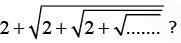Solution: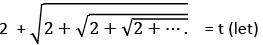2 + √t = t
Or t-2 = √t
Squaring both sides
t = t2 – 4t + 4
or t2 – 5t + 4 = 0
Or t = 4,1 Now t cannot be equal to 1 as it is clear that it is always greater than 2
So t = 4

QUESTION: 22

In an examination, 52% candidates failed in English and 42% failed in Mathematics. If 17% failed in both the subjects, then what percent passed in both the subjects?

Solution: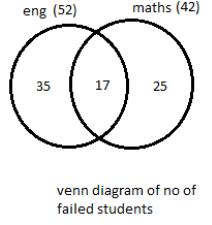No of students failed in English only = 52 – 17 = 35
No of students failed in maths only = 42 – 17 = 25
Total no of failed students in either of the subjects = 35+17+25 = 77
No of passed student in both subjects = 100 – 77 = 23

QUESTION: 23

A man who recently died left a sum of Rs. 3,90,000 to be divided among his wife, five sons and four daughters. He directed that each son should receive 3 times as much as each daughter receives and that each daughter receives and that each daughter should receive twice as much as their mother receives. What was the wife’s share?

Solution:

Let his wife get a share of Rs x
Each of the 4 daughters get = Rs 2x
Each of the 5 sons get = Rs 6x
So x + 4*2x + 5*6x = 390000
So 39x = 390000
X= 10000 = wife’s share

QUESTION: 24

What is the least number of complete years in which a sum of money put out at 40% annual compound interest will be more than trebled?

Solution:

A = P(1 + 1/R100)^t
3P < P(1 + 40/100)^t
3 < (1.4)^t
When t = 3 ; 1.4^3 = 2.744
And when t = 4; 1.4^4 = 3.8416

QUESTION: 25

A person divided a sum of Rs. 17,200 into three parts and invested at 5%. 6% and 9^ per annum simple interest. At the end of two years, he got the same interest on each part of money. What is the money invested at 9%?

Solution:

Let sum invested @ 5% be P1, @ 6% be P2 then @ 9% = 17200-(P1+P2)
So according to question
P1*5*2/100 = P2*6*2/100 or P1 = (6/5) P2
Also  P2*6*2/100 = [17200-(P1+P2)]*9*2/100
Or  2 P2 = [17200 – (11/5)P2] * 3
Or (2 + 33/5)P2 = 17200 * 3
P2 = 17200 * 3 * 5 / 43 = 6000
So P1 = 6/5 P2 = 7200
So Sum invested @ 9% = 17200 –(6000+7200) = Rs 4000

QUESTION: 26

The corners of a square of side ‘a’ are cut away so as to form a regular octagon. What is the side of the octagon?

Solution: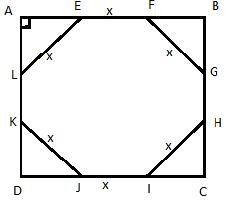Let side of hexagon be x
AE2 + AL2 = LE2
Since we are forming a regular octagon so AE = AL = FB = BG and so on
So AE = SB = x/√2
AE + EF + FB = side of square = a (Given)
So x/√2 + x + x/√2 = a
X = a/(√2+1) = a(√2 - 1)

QUESTION: 27

Three consecutive integers form the lengths of a right-angled triangle. How many sets of such three consecutive integers is/are possible?

Solution:

let n-1, n, n+1 be 3 consecutive integers
So
(n+1)2 = n2 + (n-1)2
(n+1)2- (n-1)2 = n2
4n = n2
So n = 0 or n = 4
n can’t be 0 as n-1 will be negative then
So 3,4 and 5 is the only triplet formed

QUESTION: 28

Two circles are drawn with the same centre. The circumference of the smaller circle is 44 cm and that of the bigger circle is double the smaller one. What is the area between these two circles?

Solution: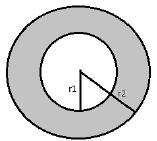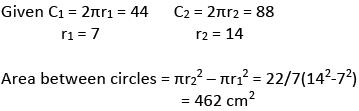QUESTION: 29

A rectangular red carpet of size 6 ft × 12 ft has a dark red border 6 inches wide. What is the area of the dark red border?

Solution:

Initially carpet is 6×12 = 72 sq feet
Since red border is 6 inches wide from all 4 side
So area without border = 5 × 11 = 55 sq feet
Area of border = total – area without border = 72 – 55 = 17 sq feet

QUESTION: 30

The perimeter of a right-angled triangle is k times the shortest side. If the ratio of the other side to hypotenuse is 4 : 6, then what is the value of k?

Solution:

Let other side and hypotenuse be 4x and 5x respectively
Shortest side2 + (4x)2 = (5x)2
Shortest side = 3x
According to question
K*3x = 12x
So k = 4

QUESTION: 31

A 12 m long wire is cut into two pieces, one of which is bent into a circle and the other into a square enclosing the circle. What is the radius of the circle?

Solution: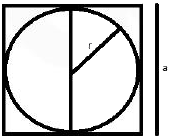As it is clear that 2r = a where a is the side of the square and R is the radius of circle
It is given that 2πr + 4a = 12
a = 12/(π+4)

QUESTION: 32

The angles of a triangle are in the ratio 1 : 1 : 4. If the perimeter of the triangle is k times its largest side, then what is the value of k?

Solution:

4k + k + k = 6x =180 degrees
k= 30 degrees
So triangle is 30,30 and 120 degrees
Let sides of triangle be x,x and y units with y being the largest side opposite to 120 degree angle
Using cosine law
Cos 120 = - sin 30 = -1/2 = (2x2 – y2)/2x2
So 3x2 = y2 …. (i)
Given Perimeter = k (Largest side)
Or 2x+y = ky
Putting value of x from eq (i)
2y/√3 + y = ky
K = 2/√3 + 1

QUESTION: 33

The hypotenuse of a right-angled triangle 10 cm and its area is 24 cm2. If the shorts side is halved and the longer side is double, the new hypotenuse becomes

Solution:

Hypotenuse = 10cm
Let the other 2 perpendicular sides be a and b
Area ½ a*b = 24
So a*b = 48 cm2
Also using Pythagoras
a2 + b2 = 100
(a+b)2 = a2 + b2 + 2ab = 100 + 96 = 196
a+b = 14
Similarly
a-b = 2
So
a=8 and b=6
Now smaller side is halved and larger side is doubled
So a1 = 16 and b1 = 3
New hypotenuse = √(162+32) = √265

QUESTION: 34

In a circle of radius 8 cm, AB and AC are two chords such that AB = AC = 12 cm. What is the length of chord BC?

Solution: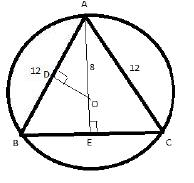O is the center of circle
Here ABC forms an isosceles triangle as AB=AC=12cm
So AE (a perpendicular bisector) passes through O as OE also bisects chord BC at right angle
OD = √64 – 36 = √28
AB/AO = EB/DO
12/8 = (BC/2)/ √28
BC=6√7

QUESTION: 35

Consider the following statements:
1) An isosceles trapezium is always cyclic.
2) Any cyclic parallelogram is a rectangle.
Which of the above statements is/are correct?

Solution: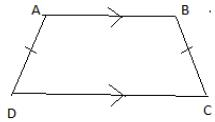Since it is an isosceles trapezium
So angle C = angle D = x let
A = 180 – D = 180 – x (since AB is parallel to CD)
B = 180-x
A+C = 180 –x + x = 180 degrees (Property of cyclic quadrilateral)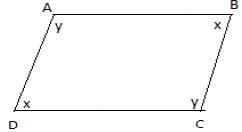ABCD is cyclic parallelogram with AB // CD and AD // BC Considering angles
A = C = y (Property of parallelogram) and B=D = x
Also since it is cyclic
A+C = B+D = 180degrees
So x=y=90degrees
And also opposite sides are equal being a parallelogram
Thus ABCD is a rectangle

QUESTION: 36

A ladder is resting against a vertical wall and its bottom is 2.5 m away from the wall. If it slips 0.8 m down the wall, then its bottom will move away from the wall by 1.4 m. What is the length of the ladder?

Solution: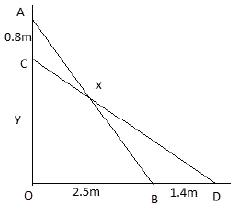AB = CD = x = Length of ladder
Let OC = y m
y2 + 3.92 = x2
(y+0.8)2 + 2.52 = x2
So y2 + 3.92 = (y+0.8)2 + 2.52
y = 5.2m
x= √(5.22+3.92)
x= 6.5m

QUESTION: 37

Two equal circles intersect such that each passes through the centre of the other. If the length of the common chord of the circles is 10√3 cm, then what is the diameter of the circle?

Solution: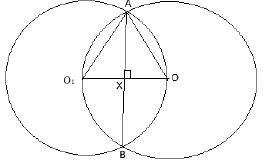Let there be 2 circles with centre O1 and O
AB is the common chord
Since both passes through the center of each other as shown in figure
So O1O is the radius of both Let O1O = r = AO1 = AO
AX = AB / 2 = 5√3 cm (since OX perpendicular to chord bisects it)
AOO1 forms an equilateral triangle with on side = radius = r
Sin 60 = √3/2 = AX / AO = 5√3/r
So r = 10cm
So diameter = 20 cm

QUESTION: 38

Consider the following statements:
1) The number of circles that can be drawn through three non-collinear points is infinity.
2) Angle formed in minor segment of a circle is acute.
Which of the above statements is/are correct?

Solution:

(1) Only one circle can be drawn through 3 non collinear points
Angle in the minor segment is always obtuse

QUESTION: 39

Consider the following inequalities in respect of any triangle ABC:
1) AC – AB < BC
2) BC – AC < AB
3) AB – BC < AC
Which of the above are correct?

Solution:

AC-AB<BC Or AB+BC>AC
BC-AC<AB Or AB+AC>BC
AB-BC<AC Or AC+BC>AB
Sum of 2 sides of triangle is always greater than the third side
So all three statements are true

QUESTION: 40

Consider the following statements:
1) The perimeter of a triangle is greater than the sum of its three medinas.
2) In any triangle ABC, if D is any point on BC, then AB + BC + CA > 2AD.
Which of the above statements is/are correct?

Solution:

1. Perimeter of triangle is greater than the sum of 3 medians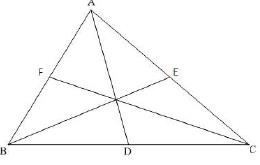Let ABC be the triangle and D. E and F are midpoints of BC, CA and AB respectively.
Recall that the sum of two sides of a triangle is greater than twice the median bisecting the third side,(Theorem to be remembered)
Hence in ΔABD, AD is a median
⇒ AB + AC > 2(AD)
Similarly, we get
BC + AC > 2CF
BC + AB > 2BE
On adding the above inequations, we get
(AB + AC) + (BC + AC) + (BC + AB )> 2AD + 2CD + 2BE
2(AB + BC + AC) > 2(AD + BE + CF)
∴ AB + BC + AC > AD + BE + CF
2.
To prove: AB + BC + CA > 2AD
Proof: In triangle ABD, AB + BD > AD [because, the sum of any two sides of a triangle is always greater than the third side] ---- 1
In triangle ADC, AC + DC > AD [because, the sum of any two sides of a triangle is always greater than the third side] ---- 2
Adding 1 and 2 we get,
=> AB + (BD + DC) + AC > 2AD
=> AB + BC + AC > 2AD
Hence proved

QUESTION: 41

Consider the following grouped frequency distribution: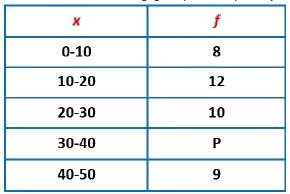If the mean of the above data is 25.2, then what is the value of p?

Solution:

Mean = (sum of fixi )/ (sum of f) = (8*5 + 12*15 + 10*25 + P*35 + 9*45) / (8+12+10+P+9) = 25.2
(875 + 35P)/(39+P) = 25.2
P = 11

QUESTION: 42

Consider the following frequency distribution: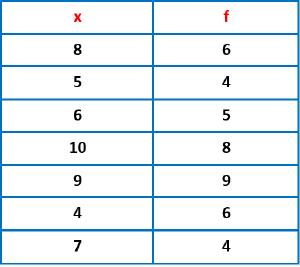What is the median for the distribution?

Solution:

Summation of frequencies = 6+4+5+8+9+6+4 = 42
Median = mid value = average of 21st and 22nd value
Arranging data in increasing order we get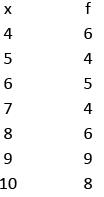So mid value i.e 21st and 22nd value  = 8

QUESTION: 43

The average of 50 consecutive natural numbers is x. What will be the are average when the next four natural numbers are also included?

Solution:

Sum of n consecutive natural numbers = n(n+1)/2
Average of n consecutive natural numbers = (n+1)/2
For first 50 average = 51/2 = x
Last 50 average = 55/2 = x+2

QUESTION: 44

Consider two-digit numbers which remain the same when the digits interchange their positions. What is the average of such two-digit numbers?

Solution:

All such 2 digit numbers are 11,22,33,44……. upto 99
Forms an AP
So sum = n/2(a+l)
= 9/2(11+99)
Average = sum/9 = ½(11+99) = 55

QUESTION: 45

Diagrammatic representation of data includes which of the following?
1) Bar diagram
2) Pie-diagram
3) Pictogram
Select the correct answer using the code given below:

Solution:

All three are types of data representation
Pictogram uses pictures so show different identities with different numbers

QUESTION: 46

The data collected from which one of the following methods is not a primary data?

Solution:

Primary data is information that you collect specifically for the purpose of your research project. An advantage of primary data is that it is specifically tailored to your research needs. A disadvantage is that it is expensive to obtain.

QUESTION: 47

The monthly expenditure of a person is Rs. 6000. The distribution of expenditure on various items is as follows: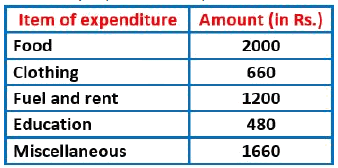If the above data is represented by a percentage bar diagram of height 15 cm, then what are the lengths of the two segments of the bar diagram corresponding to education and miscellaneous respectively?

Solution:

15 cm corresponds to 6000 rs
Education = 480/6000 * 15 cm = 1.2cm
Miscellaneous = 1660/6000 * 15cm = 4.15 cm

QUESTION: 48

If the mean of m observations out of n observations is n and the mean of remaining observations is m, then what is the mean of all n observations?

Solution:

Mean of m observations is n
Mean of n-m observations is m
So total =  nm + (n-m)m
Total observations = n
Mean = Total / Total observations = (2mn-m2)/n = 2m – m2/n

QUESTION: 49

Which one of the following pairs is correctly matched?

Solution:

An ogive (oh-jive), sometimes called a cumulative frequency polygon, is a type of frequency polygon that shows cumulative frequencies. In other words, the cumulative percents are added on the graph from left to right. An ogive graph plots cumulative frequency on the y-axis and class boundaries along the xaxis. Only median can be traced using frequency polygon curve. Thus it has a graphical location on the curve. Hence the only option correctly matched is option A.

QUESTION: 50

The following pairs relate to frequency distribution of a discrete variable and its frequency polygon. Which one of the following pairs is not correctly matched?

Solution:

Area of the polygon gives sum of fixi not summation of fi

QUESTION: 51

In a rectangle, length is three times its breadth. If the length and the breadth of the rectangle are increased by 30% and 100% respectively, then its perimeter increases by

Solution:

Let the breadth of the rectangle = x Length of the the rectangle will be = 3 times of breadth = 3x
So the initial perimeter = 2(length + breadth) = 2(x + 3x) = 8x
New breadth after increase = x + 10x/100 = 1.1x
New length after increase = 3x + 30*3x/100 = 3.9x
New perimeter = 2(1.1x + 3.9x) = 10x
Percentage change in perimeter = ( 10x-8x )*100/8x = 25%

QUESTION: 52

What is the percentage decrease in the area of a triangle if its each side is halved?

Solution:

Area of triangle of = ½*a*b* sinθ = A
Where a and b are sides of the triangle and θ be the angle between them
After decreasing each side
New area = ½*(a/2)*(b/2)*sinθ = ¼ A
%decrease = [(A – ¼ A)/A ]*100 = 75%

QUESTION: 53

The volume of a spherical balloon is increased by 700%. What is the percentage increase in its surface area?

Solution:

Let the volume of spherical balloon initially = V
New volume after increase = V + 700*V/100 = 8V
Since we know that volume of sphere is directly proportional to the radius of sphere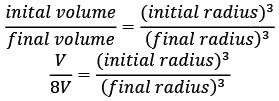Since surface area of sphere is directly proportional to the square of the radius of sphere,()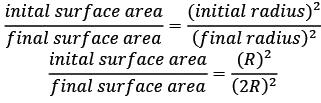Final surface area = 4*initial surface area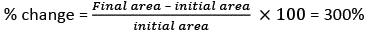QUESTION: 54

If the lengths of two parallel chords in a circle of radius 10 cm are 12 cm and 16 cm, then what is the distance between these two chords?

Solution: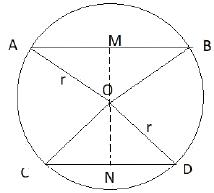Case – 1 When both the chords are in two different halves of the circle
Distance between chords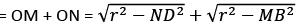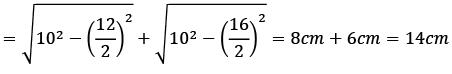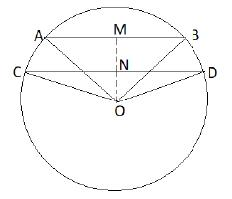Case – 2 When both the chords are in two different halves of the circle
Distance between chords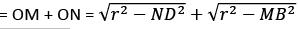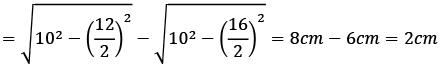QUESTION: 55

Considering two opposite vertices of a square of side ‘a’ as centres, two circular arcs are drawn within the square joining the other two vertices, thus forming two sectors. What is the common area in these two sectors?

Solution: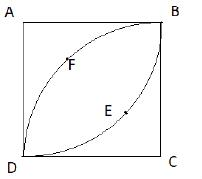Area of leaf BEDFB = Area of two quarter circle – area of square
= 2πr2/4 – a2
= πa2/2 – a2 = a2(π/2 - 1)

QUESTION: 56

What is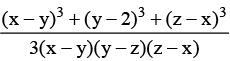equal to?

Solution:

We know that when a+b+c = 0, then
a3 + b3 + c3 = 3abc
in the above question,
(x-y) + (y-z) + (z-x) = 0
Therefore,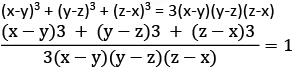QUESTION: 57

If ax = by = cz and b2 = ac, then what is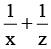equal to?

Solution:

ax = by = cz = k
a = k1/x
b = k1/y
c = k1/z
given b2 = ac, putting the above values of a,b,c in the equation we get
k2/y = k1/x .k1/z
2/y = 1/x + 1/z

QUESTION: 58

If p and q are the roots of the equation x2 – 15x + r = 0 and p – q = 1, then what is the value of r ?

Solution:

In the below equation,
x2 – 15x + r = 0
sum of roots = p + q = -(-15)/1 = 15 (sum of roots for equation ax2 + bx + c is -b/a)
product of roots = pq = r/1 = r (product of roots for equation ax2 + bx + c is c/a)
given p – q = 1
also we know that p+q = 15
subtracting the squares of both
(p+q)2 + (p-q)2 = 152 – 1
p2 + q2 + 2pq – p2 – q2 +2pq = 225 -1
4pq = 224
4r = 224
r = 56

QUESTION: 59

For the inequation x2 – 7x + 12 > 0, which one of the following is correct ?

Solution: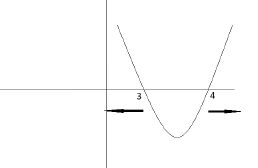As we can see from the graph of the quadratic equation, that the value of the equation is greater than zero for the values of  x < 3 and x > 4

QUESTION: 60

The expression 52n – 23n has a factor?

Solution:

52n – 23n = ( 52 )n – ( 23 )n = (25)n – (8)n
We know that an – bn always have a common factor (a - b)
Therefore one of the factor is  25 – 8 = 17

QUESTION: 61

If tan x = 1, 0 < x < 90o, then what is the value of 2 sin x cos x?

Solution:

tan x = 1
then
x = 45o
2sin x. cos x =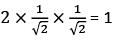QUESTION: 62

What is the value of sin 46o cos 44o + cos 46o sin 44o?

Solution:

sin 46o. cos 44o + cos 46o. sin 44o
sin 46o. sin (90 - 44)o + cos 46o. cos (90 - 44)o
= sin2 46o + cos2 46o = 1

QUESTION: 63

Suppose 0 < θ < 90o, then for every θ 4 sin2 θ + 1 is greater than or equal to ?

Solution:

We know that,
Arithmetic mean ≥ Geometric mean
(4sin2 θ + 1)/2 ≥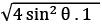4sin2 θ + 1 ≥ 2. 2 sin θ
4sin2 θ + 1 ≥  4sin θ

QUESTION: 64

Consider a regular hexagon ABCDEF. Two towers are situated at B and C. The angle of elevation from A to the top of the tower at B is 30o, and the angle of elevation to the top of the tower at C is 45o. What is the ratio of the height of towers at B and C?

Solution: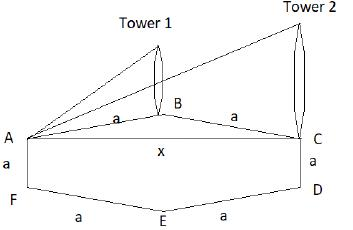Let the side of regular hexagon be ‘a’
Let height of the tower1 be h1 and tower 2 be h2
Height of tower 1 = h1 = (distance between A and B)* (tan 30o) =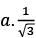Distance between A and C =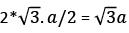Height of tower 2 = h2 = (distance between A and C)* (tan 45o) =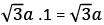Ratio of height of towers at B and C respectively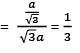QUESTION: 65

What is the value of tan 1o tan 2o tan 3o ……. Tan 89o?

Solution:

tan 1o. tan 89o = tan 1o. cot 1o = 1
similarly,
tan 2o. tan 88o = tan 2o. cot 2o = 1
tan 3o. tan 87o = tan 3o. cot 3o = 1
hence the equation will reduce to
tan 45o = 1

QUESTION: 66

There are two parallel streets each directed north to south. A person in the first street travelling from south to north wishes to take the second street which is on his right side. At some place, he makes a 150o turn to the right and he travels for 15 minutes at the speed of 20 km/hr. After that he takes a left turn of 60o and travels for 20 minutes at the speed of 30 km/hr in order to meet the second street. What is the distance between the two streets?

Solution: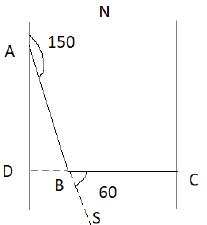Initially the person is travelling from south to north i.e. D to A
He takes 150o right turn and moves AB distance and then he takes 60o left turn travels BC
AB = 20km/hr * 15/60 hr = 5km
BC = 30 * 20/60 = 10 km
We know that distance between both the streets is DC = DB + BC
DB = AB cos 60o = 5. ½ = 2.5 km
So the distance between streets = 12.5 km

QUESTION: 67

If 3 tan θ = cot θ where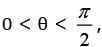then what is the value of θ?

Solution:

3tan θ = cot θ
3tan θ = 1/tan θ
tan2 θ = 1/3
tan θ = 1/√3
θ = π/6

QUESTION: 68

What is the value of sin2 25o + sin2 65o?

Solution:

sin2 25o + sin2 65o = sin2 25o + sin2 (90 – 25)o = sin2 25o + cos2 25o = 1

QUESTION: 69

What is the value of sin6 θ + cos6 θ + 3 sin2 θ cos2 θ – 1?

Solution:

sin6 θ + cos6 θ + 3sin2 θ .cos2 θ - 1
sin6 θ + cos6 θ + 3sin2 θ .cos2 θ. 1 - 1
sin6 θ + cos6 θ + 3sin2 θ .cos2 θ. (sin2 θ + cos2 θ ) - 1
(sin2 θ + cos2 θ )3 – 1 = 1 – 1 =0

QUESTION: 70

Consider the following for real numbers α, β, γ and δ?
1) sec α = 1/4
2) tan β = 20
3) cosec γ = 1/2
4) cos δ = 2
How many of the above statements are not possible?

Solution:

Sec of any number can never be less than 1
tan can take any value from -∞ to + ∞
cosec of any number can never be less than 1
cos of any number can never be greater than 1
so option 1,3,4 are not possible

QUESTION: 71

Direction: Consider the following for the next three (03) items:
In a certain town of population size 1,00,000 three types of newspapers (I, II and III) are available.
The percentages of the people in the town who read these papers are as follows: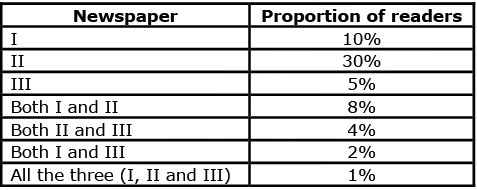What is the number of people who read only one newspaper?

Solution: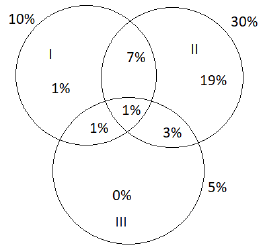The number of people who read only I , only II and only II are
1 % + 19% + 0% = 20% of total population = 20/100 * 100000 = 20000

QUESTION: 72

Direction: Consider the following for the next three (03) items:
In a certain town of population size 1,00,000 three types of newspapers (I, II and III) are available.
The percentages of the people in the town who read these papers are as follows: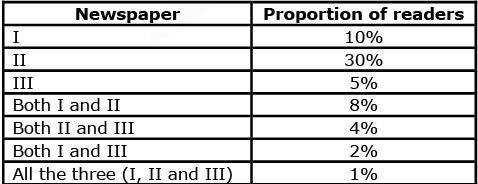What is the number of people who read at least two newspaper?

Solution: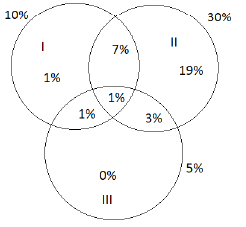As we can see from the above venn diagram the number of people who read two or more newspapers are 1% + 1% + 3% + 7% = 12% = 12/100 * 100000 = 12000

QUESTION: 73

Direction: Consider the following for the next three (03) items:
In a certain town of population size 1,00,000 three types of newspapers (I, II and III) are available.
The percentages of the people in the town who read these papers are as follows: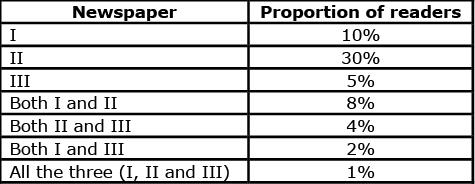What is the number of people who do not read any of these three newspapers?

Solution: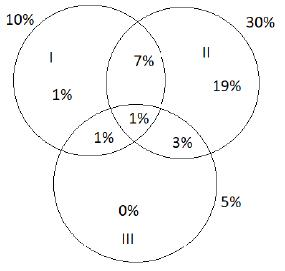Number of people who do not read any of these newspaper = total population – number of people who read atleast one of these newspapers.
number of people who read atleast one of these newspapers = 1% + 1% + 3% + 1% + 7% + 19% = 32% of total population = 32000
required number of people = 100000 – 32000 = 68000

QUESTION: 74

What is the unit place digit in the expansion of 773?

Solution: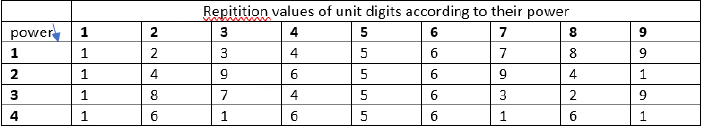From the above table we can see that the power 73 is of the form 4x + 1
Therefore the unit digit according to the table = 7

QUESTION: 75

Suppose n is a positive integer such that (n2 + 48) is a perfect square. What is the number of such n?

Solution:

N2 + 48 =k2
48 =k2 – N2
(k - N)(k + N) = 48
So the possible number of pairs of (k - N) and (k + N) are
(1,48),(2,24), (3,16), (4,12), (6,8)
On solving the above pairs for (k - N) and (k + N), we get the integer values of N and k as
N=1 ,k= 7
N=4 , k=8
N=11,k=13
So the total possible values of N are three

QUESTION: 76

For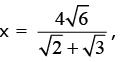what is the value of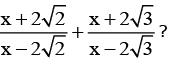Solution: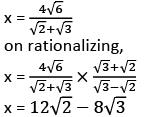putting the value of x in the equation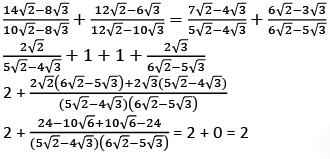QUESTION: 77

x, y and z are three numbers such that x is 30% of z and y is 40% of z. If x is p% of y, then what is the value of p?

Solution:

x = 30% of z = 30z/100 = 3z/10
y = 40% of z = 40z/100 = 4z/10
According to the question,
(x/y)*100 = p%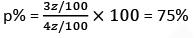QUESTION: 78

A plane is going in circles around an airport. The plane takes 3 minutes to complete one round. The angle of elevation of the plane from a point P on the ground at time t seconds is equal to that at time (t + 30) seconds. At time (t + x) seconds, the plane flies vertically above the point P. What is x equal to?​

Solution: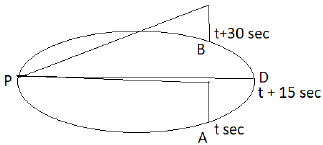Let the plane be at point A at t seconds and at point B after t + 30 seconds
Since the motion is uniform, we can say that at time t+15 seconds, the plane is above the point is diametrically opposite to the point P from where the angle is same.
Now since the time taken to cover the full circle is 3 minutes (180 seconds), the time taken by the plane to reach the diametrically opposite point will be 90 seconds.
So the time after which the plane reaches the point P will be = t+ 15 + 90 seconds =  (t + 105 )seconds

QUESTION: 79

Consider the following statements in respect of two integers p and q (both > 1) which are relatively prime:
1) Both p and q may be prime numbers.
2) Both p and q may be composite numbers
3) one of p and q may be prime and the other composite.
Which of the above statements are correct?

Solution:

All the given statements are true. The following are the examples for all the statements
Statement 1: Both p and q may be prime numbers. E.g. 3 and 5
Statement 2 : Both p and q may be composite numbers. E.g. 4 and 9
Statement 3 : One of p and q may be prime and the other composite. E.g. 7 and 12

QUESTION: 80

In a class of 100 students, the average weight is 30 kg. If the average weight of the girls is 24 kg and that of the boys is 32 kg, then what is the number of girls in the class?

Solution:

By alligation,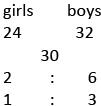So the number of girls will be =( 1/(1+3))*100 = 25

QUESTION: 81

For any two real numbers a and b.​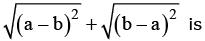Solution:

For the equation,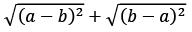Where a and b are real numbers,
The roots of number is always positive and hence it can be zero only at a=b
So the above equation is positive only when a=b

QUESTION: 82

If a : b = c : d = 1 : 6, then what is the value of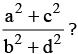Solution:

Let a = x then b = 6x
Also let c = y then d = 6y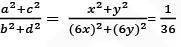QUESTION: 83

What is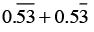equal to?

Solution: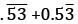= 0.5353535353….+0.5333333333…..
= 1.068686868 =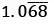QUESTION: 84

The inequality 3N > N3 holds when

Solution:

3N > N3 holds for all the natural numbers except N = 3 at which 3N = N3

QUESTION: 85

Which one of the following is an irrational number?

Solution:

A number that cannot be represented in the form p/q where p and q are two integers, is known as irrational number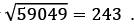Hence it is rational 231/593 is already in the form of rational number 0.4545454545……. can be represented in the form of p/q as 5/9 0.12112211122211112222……… cannot be represented in the form of p/q as there is no recurring digits in the given number

QUESTION: 86

What is the remainder when (1729 + 1929) is divided by 18?

Solution:

The number 1729 = (18 - 1)29 when divided by 18 leaves the remainder (-1)29 = 18-1 = 17
The number 1929 = (18 + 1)29 when divided by 18 leaves the remainder (1)29 = 1
Then after adding these two the remainder will be 17+1 = 18 which is divisible by 18
Hence the remainder will be 0

QUESTION: 87

What is the largest value of n such that 10n divides the product
25 × 33 × 48 × 43 × 67 × 76 × 812 × 99 × 106 × 1512 × 2014 × 2211 × 2515?

Solution:

For the number to be divisible by 10n , it must contain the same powers for 2 and 5
Power of 2 = 25+2.8+7+3.12+6+2.14 + 11 = 25+16+7+36+6+28+11 = 2109
Power of 5 = 53+6+12+14+2.15 = 565
Hence maximum possible power of 10 can be 65 only.

QUESTION: 88

How many pairs (A, B) are possible in the number 479865AB if the number is divisible by 9 and it is given that the last digit of the number is odd?

Solution:

If the number is divisible by 9 the sum of all its digit is divisible by 9
4+7+9+8+6+5+A+B = 39 + A + B is divisible by 9
Possible values of B are 1,3,5,7,9 as it is given that last digit is odd
For B= 1, A=5
For B = 3 A= 3
For B= 5 , A = 1
For B = 7, A = 8
For B = 9, A= 6

QUESTION: 89

Consider the multiplication 999 × abc = def132 in decimal notation, where a, b, c, d, e and f are digits. What are the values of a, b, c, d, e and f respectively?

Solution:

999 x abc = def132
We can write the above equation as
(1000 – 1) x abc = def132
abc000 – abc = def000 + 132 = (def +1)x 1000  - 868
on comparing the LHS and RHS, we get
a = 8, b = 6, and c = 8 and d = a = 8 , e = b = 6 and f = c – 1 = 8 -1 = 7

QUESTION: 90

Three cars A, B and C started from a point at 5 pm, 6 pm and 7 pm respectively and travelled at uniform speeds of 60 km/hr, 80 km/hr and x km/hr respectively in the same direction. If all the three met at another point at the same instant during their journey, then what is the value of x?

Solution:

Distance covered by A till 6pm = 60 km
Distance covered by A till 7 pm = 120 km
Time taken by B to catch A = 60/(80-60 ) = 3 hrs
So A and B will meet at 6pm + 3 hrs = 9pm
Since we know that all three met at the same time
The time taken by C to cover 120 km difference will be  = 9pm – 7pm = 2hrs
Therefore, (x – 60 )*2 = 120
x = 120 km/hr

QUESTION: 91

Priya’s age was a cube of an integral number (different from 1) four years ago and the square of an integral number after four years. How long should she wait so that her age becomes square of a number in the previous year and cube of a number in the next year?

Solution:

Let present age of Priya be p
p-4 = n3
p+4 = √k
since n is a no >1 on putting n= 2 we get p = 12
So p+4 = 16 which is square of an integral number thus consistent with given information
Now after how many years her age becomes such that age – 1 is a square and age + 1 is a cube
Using option if we add 14 years to the current age, we get age = 26 years
Here 25 is a square and 27 is a cube thus making 14 the correct answer.

QUESTION: 92

Which of the following statements is not true?

Solution:

Option C is incorrect as 6n – 1 form can be a prime number but it is not necessarily true.
Example 35 is of form 6n-1 but is not a prime number

QUESTION: 93

For x > 0, what is the minimum value of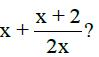Solution:

For x>0 Min of x + (x+2)/2x = ? x + (x+2)/2x = x + ½ + 1/x
So we have to find the minimum of x+1/x and add ½ to it
As AM>GM
So (x+1/x)/2 > √(x*1/x)
Or x + 1/x > 2
So min of x + (x+2)/2x = 2+1/2 = 5/2

QUESTION: 94

In a hostel the rent per room is increased by 20%. If the number of rooms in the hostel is also increased by 20% and the hostel is always full, then what is the percentage change in the total collection at the cash counter?

Solution:

Let initial rent be rs 10
And initial rooms be 10
So initial collection = 10*10 = Rs 100
Now new rent = 10 + 20% of 10 = 12
New no of rooms = 10 + 20% of 10 = 12
So new collection = 12*12 = 144
%change in collection = (144-100)/100*100 = 44%

QUESTION: 95

Radha and Hema are neighbors and study in the same school. Both of them use bicycles to go to school. Radha’s speed is 8 km/hr whereas Hema’s speed is 10 km/hr. Hema takes 9 minutes less than Radha to reach the school. How far is the school from the locality of Radha and Hema?

Solution:

Let the distance between be D km
Time is taken by Radha – Time taken by Hema = 9 mins
So D/8 – D/10 = 9/60 hrs
D = 6km

QUESTION: 96

Which of the following pair of numbers is the solution of the equation 3x+2 + 3–x = 10 ?

Solution:

3x+2 + 3-x = 10
Only powers of 3 that add upto 10 is
32 + 30 = 10
X+2 = 0
X= -2 solution is consistent
Or x+2 = 2 X= 0 solution is consistent
Thus x = 0, -2 are the solutions
Alternatively, we can put values from the options and check.

QUESTION: 97

It is given that log10 2= 0.301 and log10 3 = 0.477. How many digits are there in (108)10?

Solution:

No of digits in (108)10
We have to find the log of the given number with base 10 and add one to its integral part to find the no of digits
log (108)10 = 10 log 108 = 10 log(22 * 33) = 10[2log2 + 3log3]
= 10[2*0.301 + 3*0.477] = 20.33
Integral part = 20
No of digits = 20+1 = 21

QUESTION: 98

The sum of three prime numbers is 100. If one of them exceeds another by 36, then one of the numbers is

Solution:

Let the three prime numbers be x, y, y+36
x+y+y+36 = 100
x+2y = 64
2y is an even number always
We know that
Even + even = even or odd + odd = even
So x has to be even to satisfy x+2y = 64
The only even prime no is 2
Put x=2
2y = 62
Or y = 31
So the numbers are 2, 31, 67
Thus option D is the answer.

QUESTION: 99

If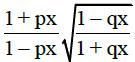= 1, then what are the non-zero solutions of x?

Solution: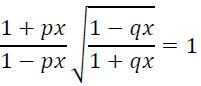On squaring and cross multiplying, we get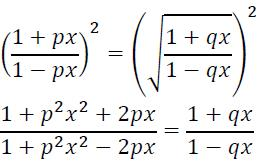On applying componendo and dividend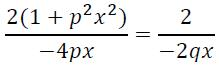On solving the above equation, we get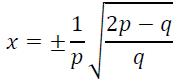QUESTION: 100

In a recent survey, 40% houses contained two or more people. Of those houses containing only one person, 25% were having only a male What is the percentage of all houses, which contain exactly one female and no males?

Solution:

Number of houses with only one female = (100 - 25)% of (100 - 40)%of x
= 75 / 100 x 60 / 100 x x
= 3 / 4 x 3 / 5 x x = 9x / 20
Required maximum percentage
= 9x / 20 x 1 / x x 100 = 45